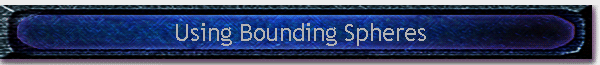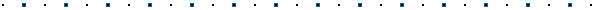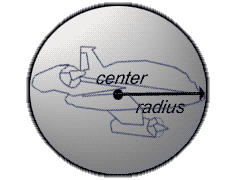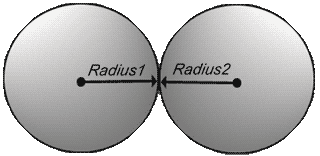#Collision Detection, Part 1: Using Bounding Spheres Written by Robert Dunlop Microsoft DirectX MVPNote Code in this article was  written for use with DirectX 8 under VC++ 6.0.  Some modification may be required for use in other development environments.## Introduction

Checking  for collision between two objects can be a very complicated task, involving  tests between the many faces of each object.  To perform this task quickly, simplified volumes are often used to represent each object, allowing fast tests for collision.  These tests are of course not as precise, and often they are used as a quick test to determine if further, detailed testing is required.

In this series of articles, we will examine three such bounding volumes:Bounding SpheresAxis Aligned Bounding BoxesOriented Bounding Boxes

We'll take a look at the strengths and weaknesses of each, as well as the code necessary to implement them.  The focus of this series will be limited to detecting an intersection.  In future articles we will take a further look at the physics behind object interaction, how to determine reactions using each of these techniques, and how to efficiently test for collisions between a collection of objects.

Now to take a look at the first of these bounding volumes, the bounding sphere.

## What is a Bounding Sphere?

 A bounding sphere is a hypothetical sphere that completely encompasses an object.  It is defined by a 3D coordinate representing the center of the sphere, and a scalar radius that defines the maximum distance from the center of the sphere to any point in the object.  A definition for a structure to specify a bounding sphere might look something like this: typedef struct _BOUNDINGSPHERE {    D3DXVECTOR3 center;    float radius; } BOUNDINGSPHERE, *LPBOUNDINGSPHERE;## Defining a Bounding Sphere

When defining a bounding sphere, one typically tries to find the tightest fit for the bounded object, that is, the smallest radius sphere that all points lie within.  Depending on the shape of the object, the techniques required for the best fit can be complex.  For simplicity sake, we'll take a look at a fairly simple algorithm that will work decently for most cases.  The function is a two step process:

1. Find the center.  In this case, we'll simply average the coordinates.
2. Loop through the coordinates and find the maximum distance from the center.  This is the radius.

To make our function versatile, I've included a stride parameter that will allow you to use this function with any structure (i.e. any conventional D3D vertex type) as long as it starts with three floats representing X, Y, and Z.  The function accepts the following parameters:A pointer to an array of coordinatesThe number of points in the arrayThe size of the structure the coordinate is contained in, in bytesA pointer to an existing BOUNDINGSPHERE structure to be filled

LPBOUNDINGSPHERE calcSphere(BYTE *vects,DWORD count,DWORD stride,LPBOUNDINGSPHERE sphere)

{

// find center

sphere->center=D3DXVECTOR3(0.0f,0.0f,0.0f);

BYTE *ptr=vects;

for (int i=0;i<count;i++) {

sphere->center+=*((LPD3DXVECTOR3) ptr);

ptr+=stride;

}

sphere->center/=(float)count;

// find farthest point in set

ptr=vects;

for (int i=0;i<count;i++) {

D3DXVECTOR3 v;

v=*((LPD3DXVECTOR3) ptr)-sphere->center;

float distSq=D3DXVec3LengthSq(&v);

ptr+=stride;

}

}

Note that we get the square of the length, and only take the square root when we have found the maximum distance.  This is to eliminate the use of a costly square root function for each point in the mesh.  You will see similar optimizations later, including the comparison of the square of a vectors length versus the square of the value we wish to compare the length to.  Multiplication is orders of magnitude faster than finding a square root.

To clarify the use of the stride, let's say we had a mesh that was composed of 1024 pre-lit vertices, that is, using the D3DLVERTEX structure.  The function would be called with the size of the D3DLVERTEX structure as the stride, like this:

// given "vects" is an array of 1024 D3DVERTEX structures

BOUNDINGSPHERE sp;

calcSphere((BYTE *) vects,1024,sizeof(D3DVERTEX),&sp);

## Determining if a Point is in a Sphere

Determining whether a given point is within a sphere is quite simple.  A surface of a sphere, just like a circle, is composed of all those points that are a constant distance (the radius) from the center point.  If the distance from a point to the center of the sphere is greater than the radius, while if it is less than the radius, the point falls within the sphere:

BOOL pointInSphere(LPD3DXVECTOR3 pt, LPBOUNDINGSPHERE sphere)

{

D3DXVECTOR3 v;

v=sphere->center-*pt;

return FALSE;

return TRUE;

}

## Determining if Two Spheres Collide

 Determining if two sphere's collide is pretty simple as well.  To gain a quick understanding of this problem, let's take a look at what happens when two spheres are touching.  As you can see in the illustration to the right, the radius of each sphere now also defines the distance its center to the opposite sphere's skin.  So, given this condition, the distance between the centers would be equal to Radius1 + Radius2.  If the distance were greater, the two spheres would not touch.  If it were less, the spheres would intersect.Below are two functions that perform this test.  The first returns a boolean value, returning TRUE if the spheres intersect or FALSE if they do not.

BOOL sphereIntersect(LPBOUNDINGSPHERE s1,LPBOUNDINGSPHERE s2)

{

D3DXVECTOR3 v;

v=s1->center-s2->center

return (D3DXVec3LengthSq(&v)<=centDist*centDist);

}

The second function returns a floating point value, which represents the distances between the closest points between the two spheres.  If the value is negative, the spheres intersect, and the value represents the greatest distance of overlap.

float sphereIntersectDist(LPBOUNDINGSPHERE s1,LPBOUNDINGSPHERE s2)

{

D3DXVECTOR3 v;

v=s1->center-s2->center;

}

## Dealing with Object Motion

So, what happens when an object moves?  Do we have to completely re-calculate the bounding sphere?  Fortunately, we do not.  Because a sphere is symmetrical, there is no change in dimensions as it is moved.  All that changes is the center point, which we must offset from model space to the current position in world space, according to the translation of the object.  Below is a class that keeps track of the origin in model space, and allows you to offset the center as needed:

class CBoundSphere
{
D3DVECTOR origin;
D3DVECTOR center;
~CBoundSphere() {};
void SetTranslation(LPD3DXVECTOR3 vOffset);
BOOL PointInSphere(LPD3DXVECTOR3 pt);
BOOL SphereIntersect(CBoundSphere *s);
float SphereIntersectDist(CBoundSphere *s);
}

{
center=origin=*vOrigin;
}

void CBoundSphere::SetTranslation(LPD3DXVECTOR3 vOffset)
{
center=origin+*vOffset;
}

BOOL CBoundSphere::PointInSphere(LPD3DXVECTOR3 pt)
{
D3DXVECTOR3 v;
v=center-pt;
}

BOOL CBoundSphere::SphereIntersect(CBoundSphere *s)
{
D3DXVECTOR3 v;
v=center-s->center;
return (D3DXVec3LengthSq(&v) <=centDist*centDist);
}

float CBoundSphere::SphereIntersectDist(CBoundSphere *s)
{
D3DXVECTOR3 v;
v=center-s->center;
}This site, created by DirectX MVP Robert Dunlop and aided by the work of other volunteers, provides a free on-line resource for DirectX programmers.

Special thanks to WWW.MVPS.ORG, for providing a permanent home for this site.

#####Visitors Since 1/1/2000:Last updated: 07/26/05.